# 7. Electronics design¶

## Group project:¶

### Use the test equipment in your lab to observe the operation of a microcontroller circuit board¶

Output whit LED that shows activation status od button.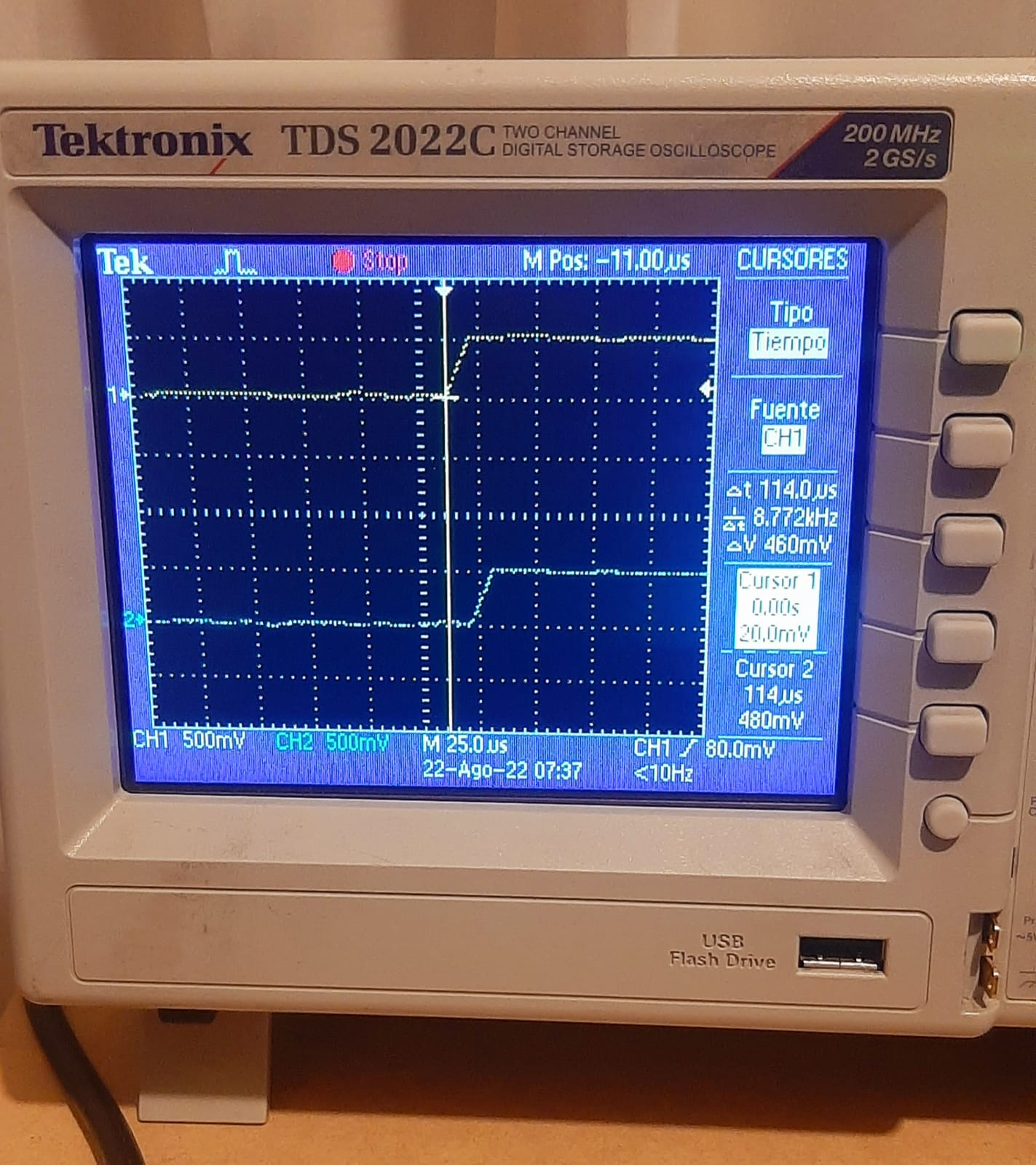Here, we show the start of button activation.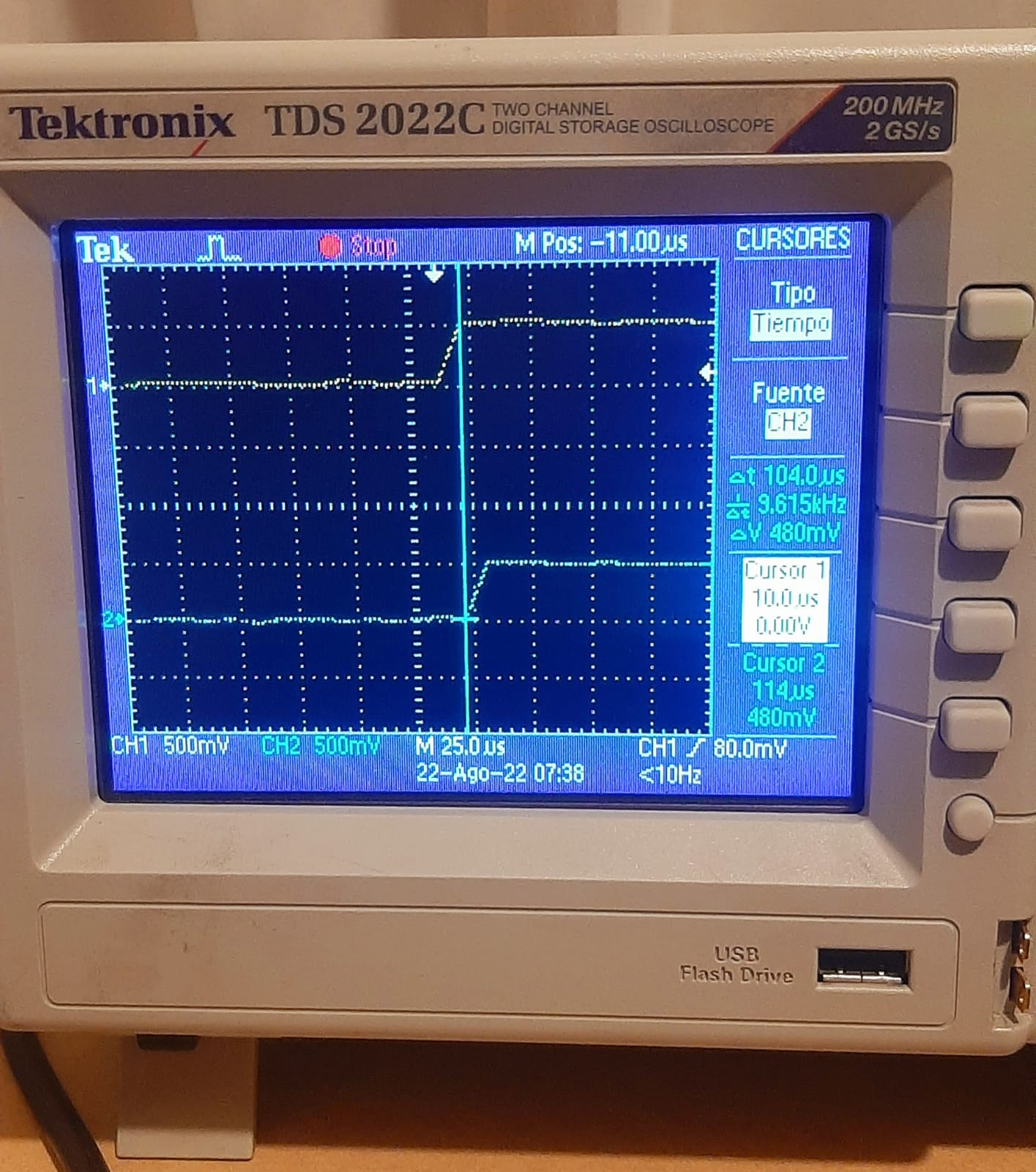Here, we show the start of pulse on output. The output pulse has 10us delay.

We show the PWM signal generated by the microcontroller.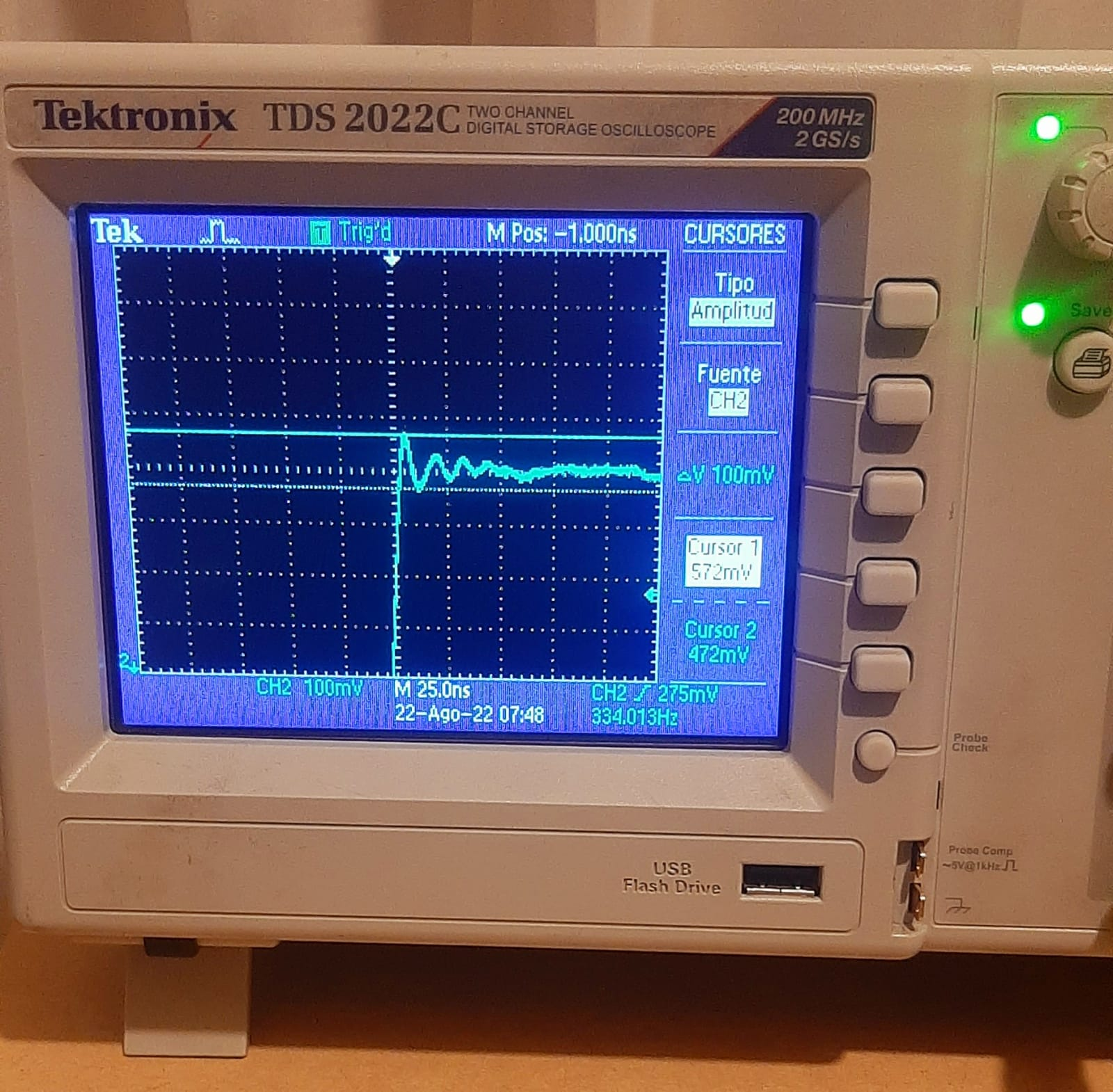Here, we show the transitory signal of the PWM pulse, we see peak-to-peak values of 100mV.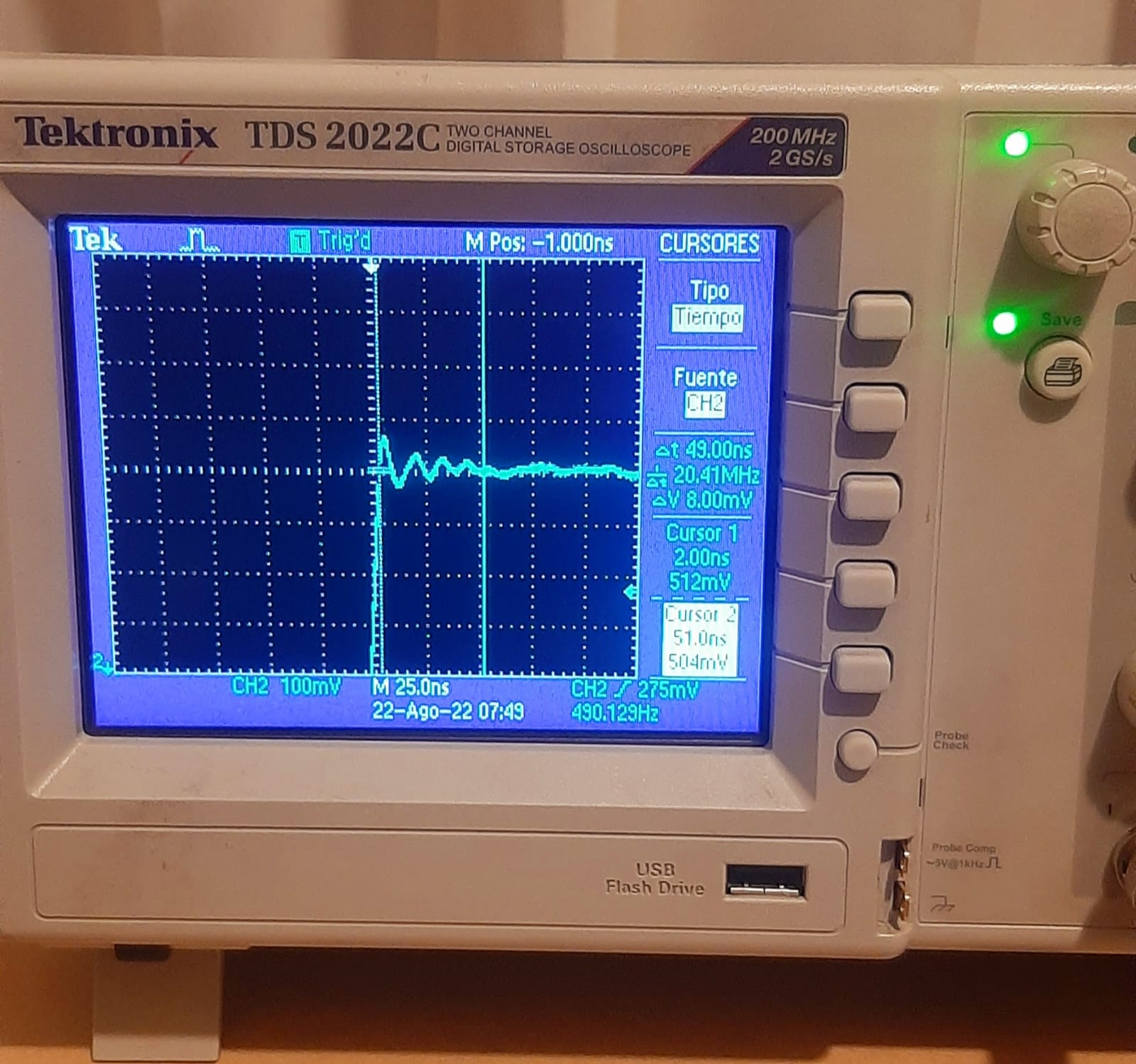Now, we see the time wide of the transitory signal, it is 49ns.

## Individual project:¶

### Redraw an echo hello-world board, add (at least) a button and LED (with current-limiting resistor) check the design rules, make it, and test that it can communicate.¶

First, I designed a general purpose circuit board with the ATmega328p microcontroller, the design was done in Eagle.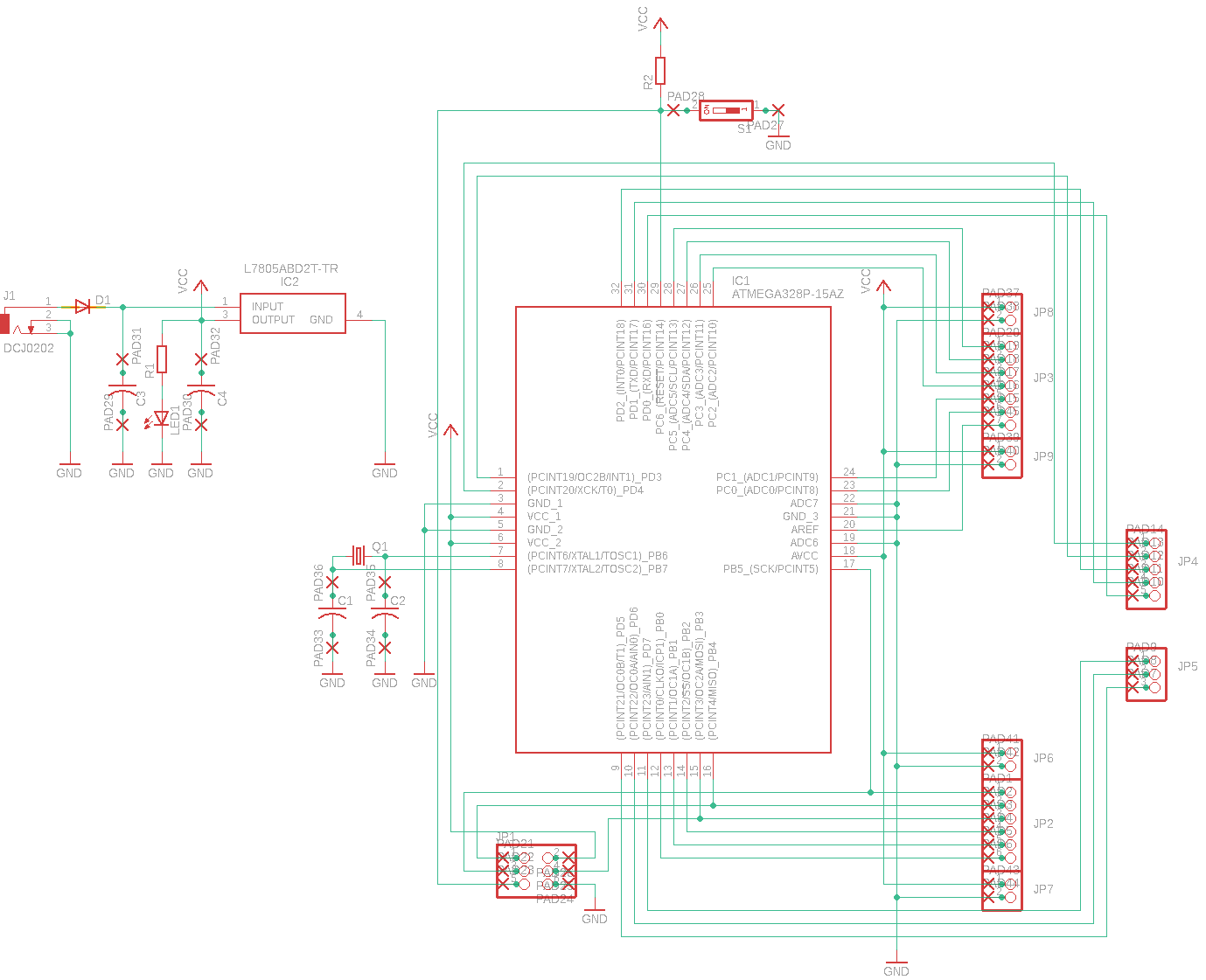Schematic circuit with ATmega328p microcontroller.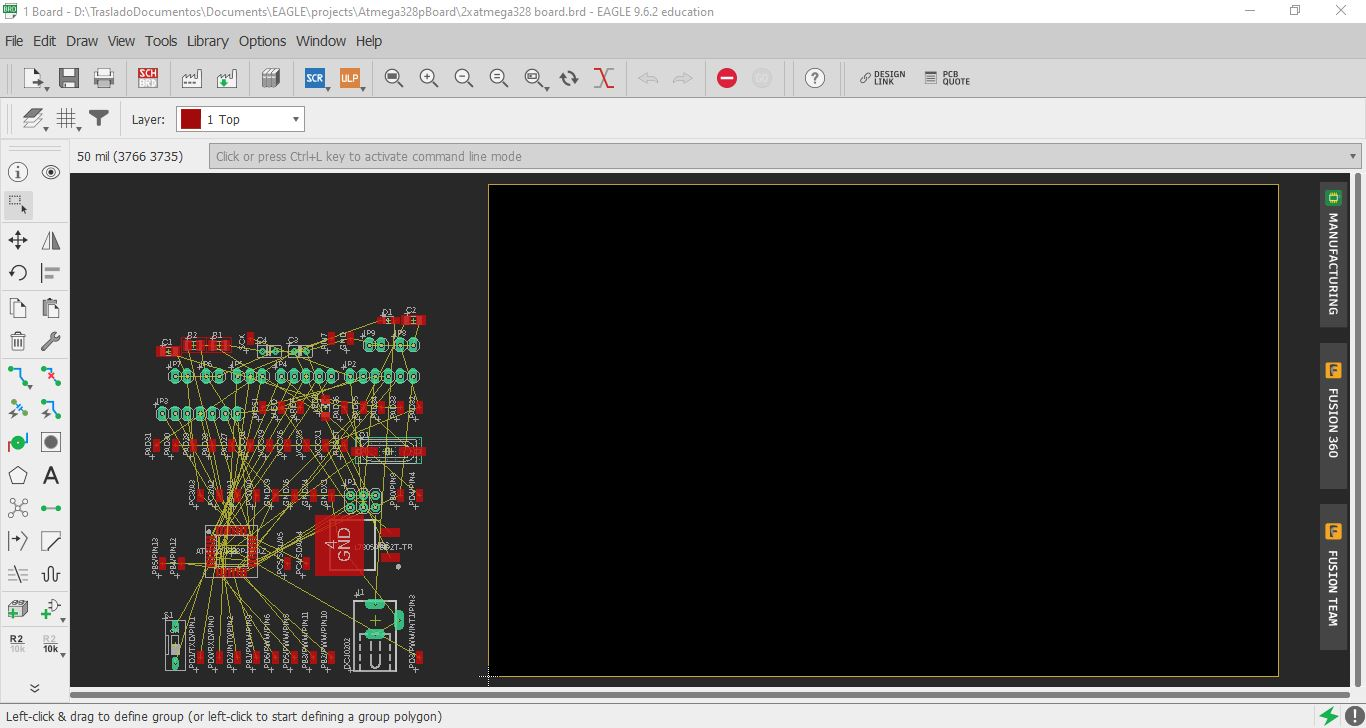We passed to schematic mode to board mode.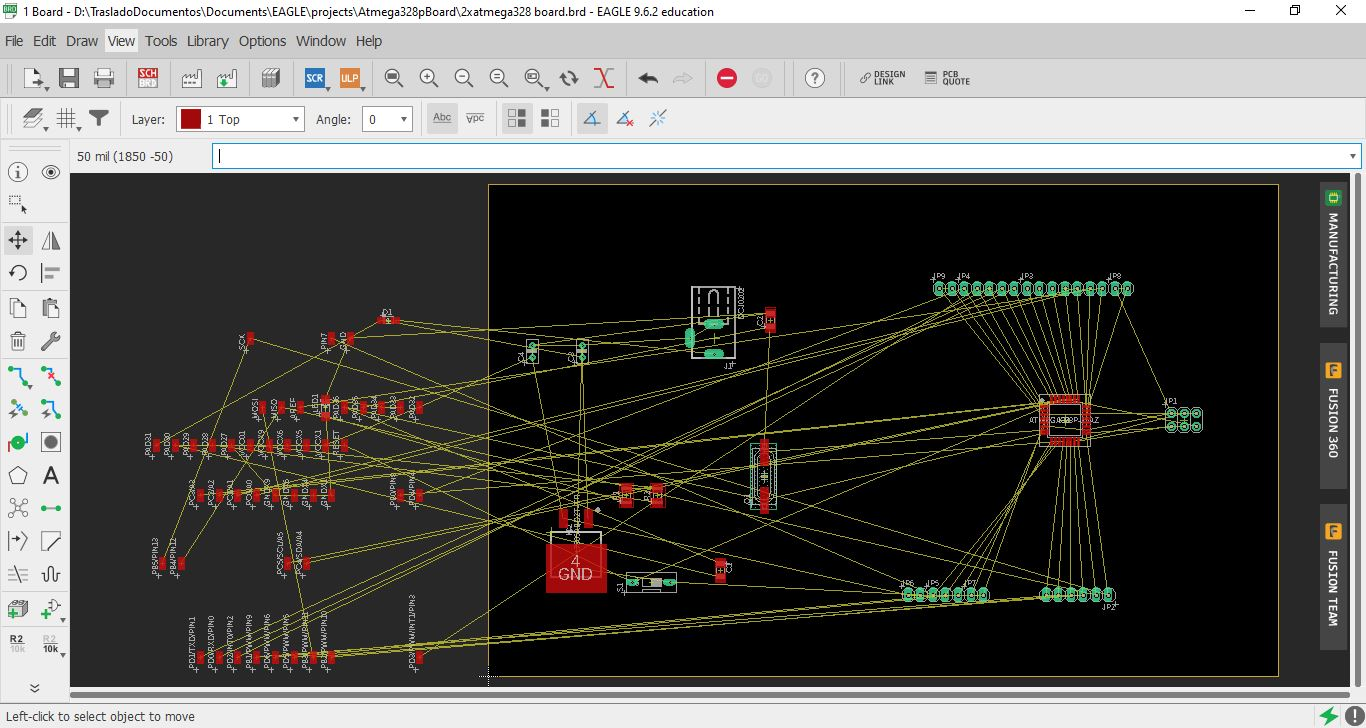We organize the location of the some components.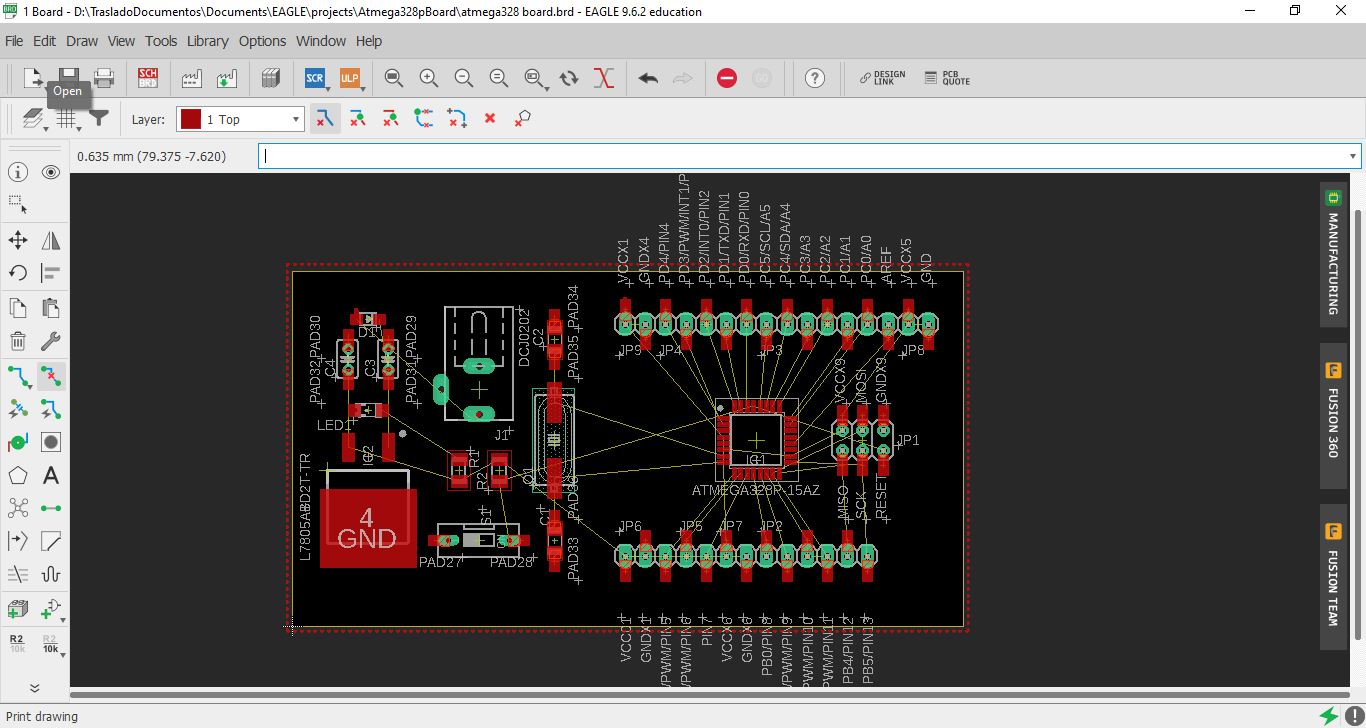We finished locating all the components.We start by connecting the microcontroller with some components.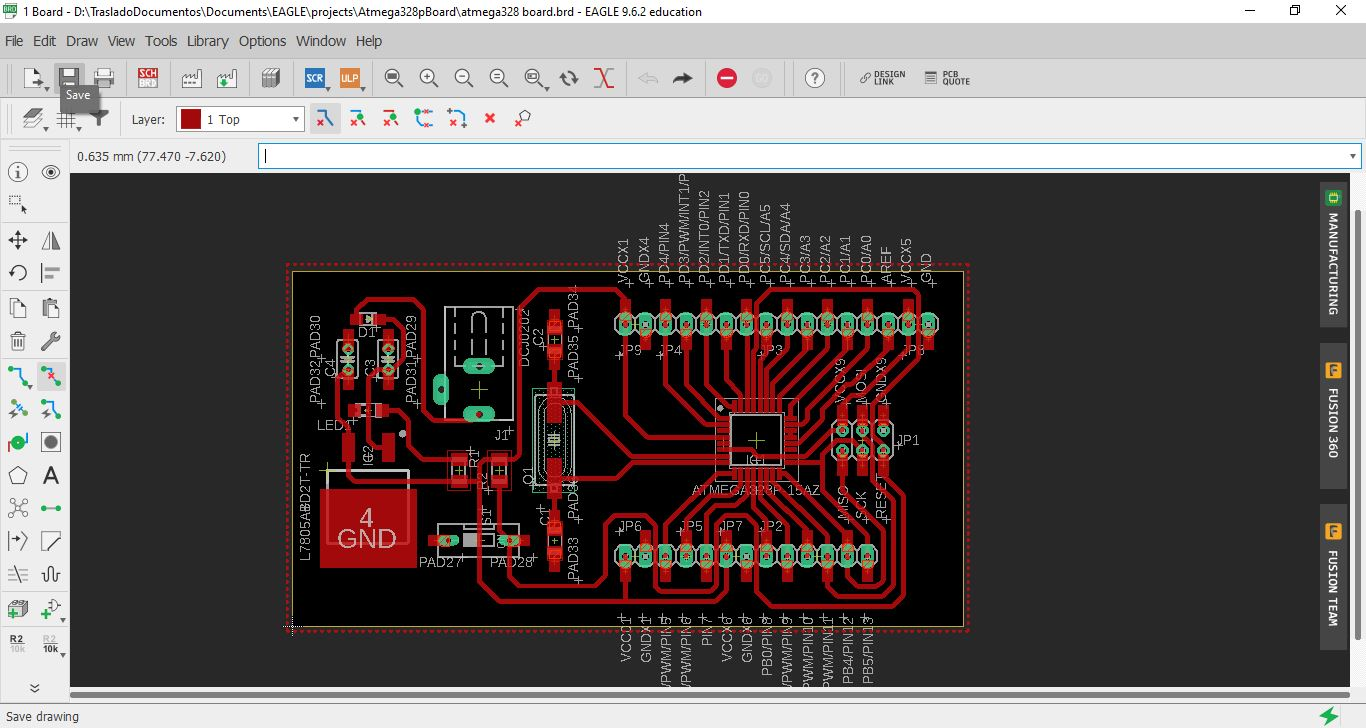We complete the connection of source and oscillator for the microcontroller.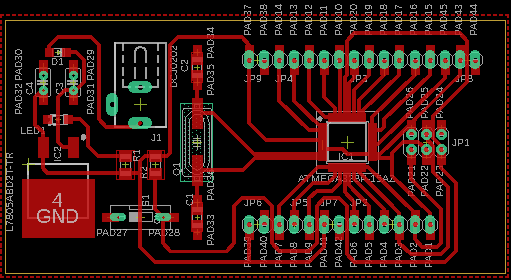Final design of the connection tracks of the components.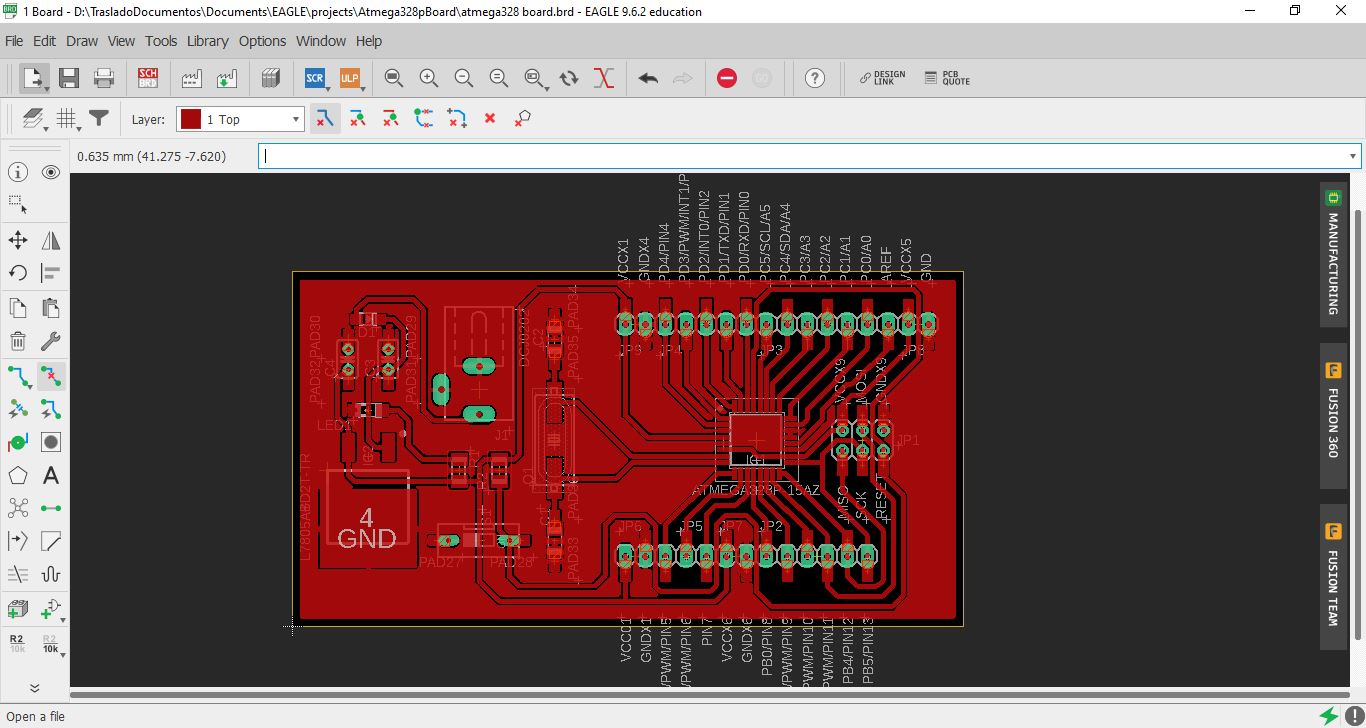Final PCB layout.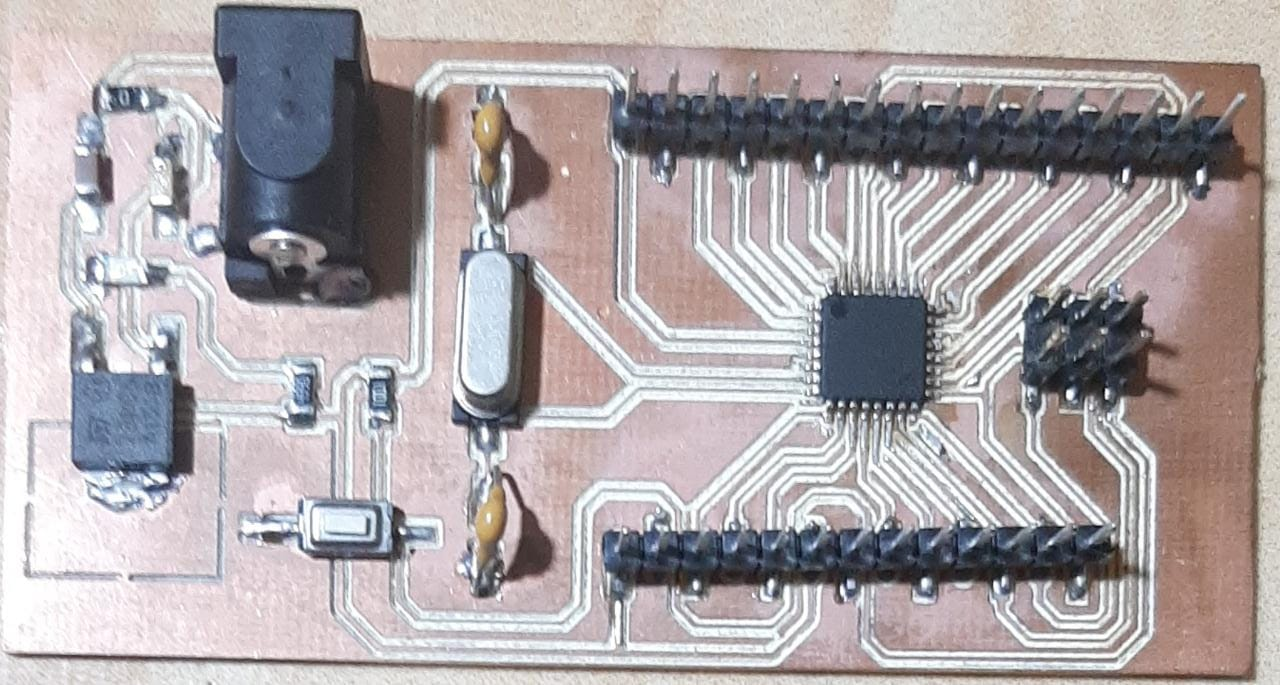PCB with the components soldered.

Next, I designed PCBs with 4 buttons.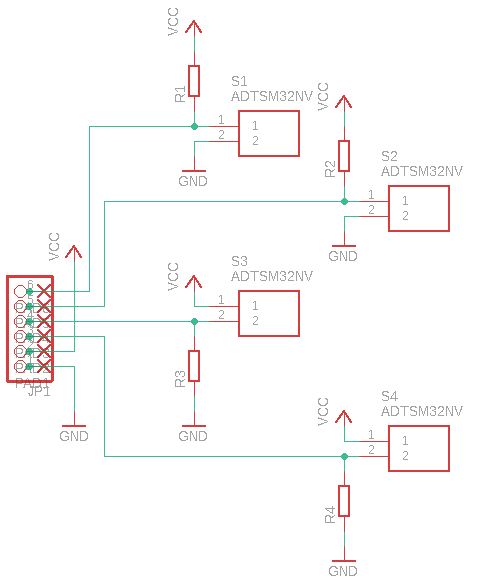Schematic circuit for four button.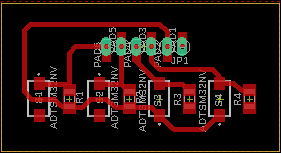PCB designed for four button.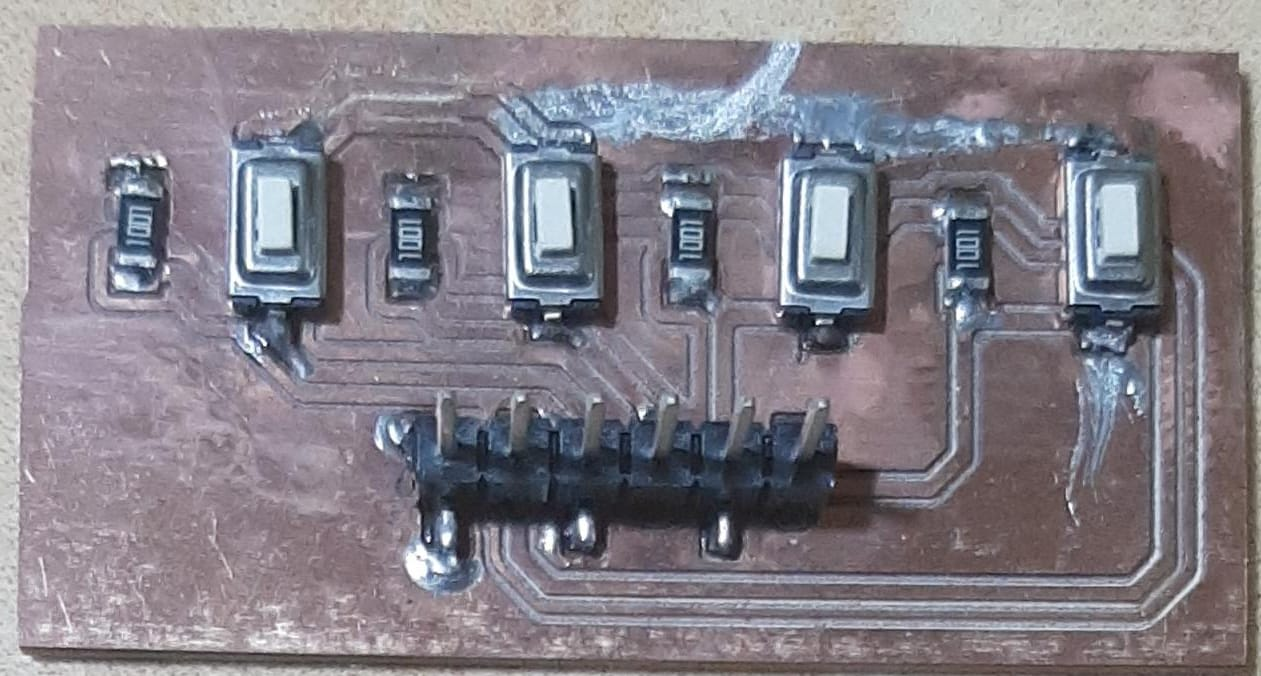PCB to four button with the components soldered.

Finally, we designed PCB with 5 leds and 5 resistors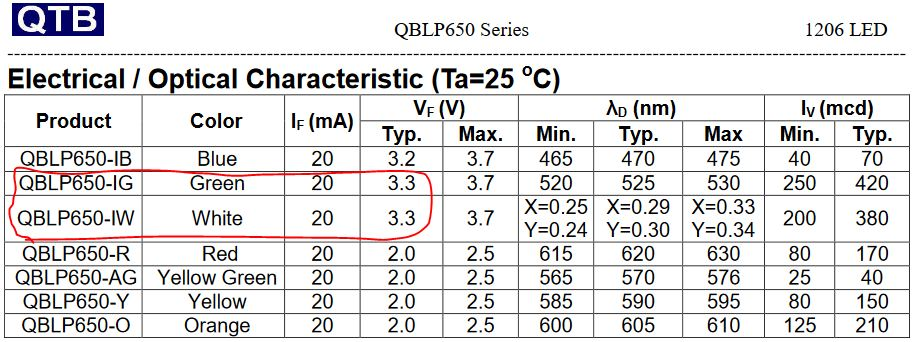The table shows the voltage drop of the green and white-yellow LEDs, this is 3.3v.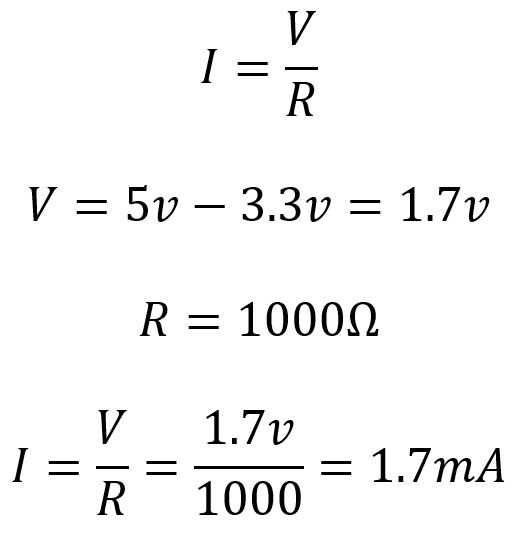Ohm’s law for current calculation, we also consider 1kΩ value resistor. We get a current of 1.7mA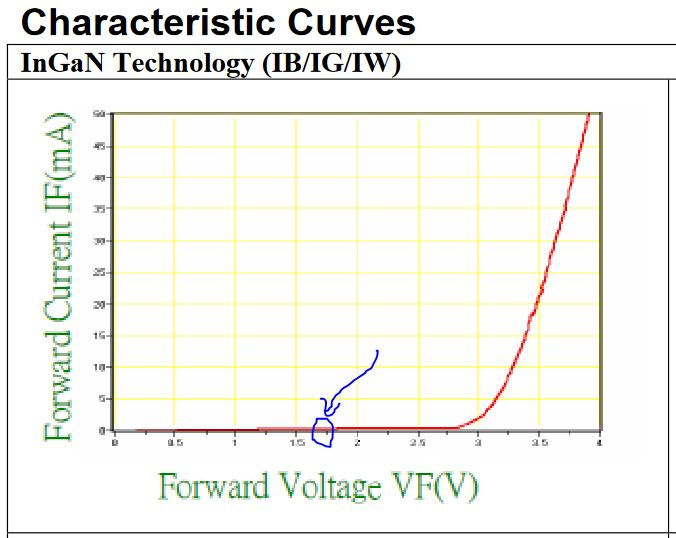We check that the calculated current is within the forward bias curve of the diode.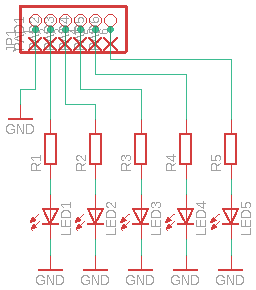Schematic circuit for five leds.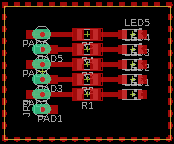PCB designed for five leds.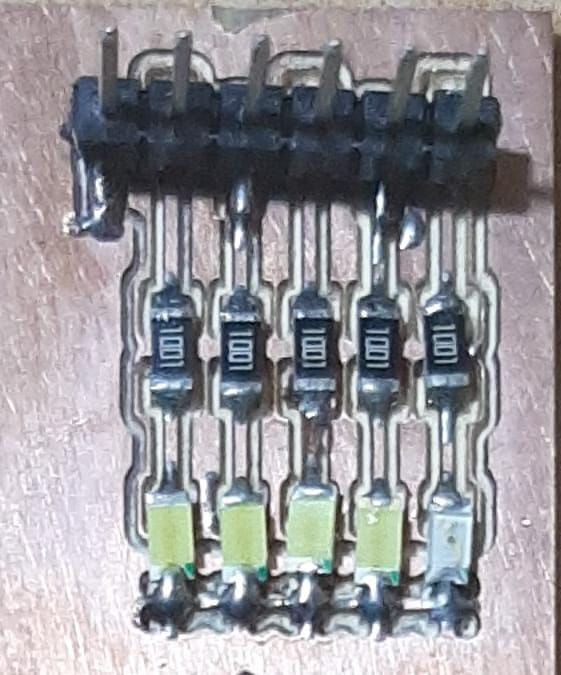PCB for five leds with the components soldered.

Code Example

``````bool PIzq = false, PDer = false, PSS = false;
int leds[] = {1, 2, 3, 4};
int pulsadores[] = {5, 6, 7};
int retardo = 250, tiempoActual, tiempoAnterior=0, j=-1;
void setup() {
// put your setup code here, to run once:
for (int i=0;i<=3;i++){
pinMode(leds[i],OUTPUT);
digitalWrite(leds[i],LOW);
}
pinMode(0,OUTPUT);
digitalWrite(0,LOW);
}

void loop() {
// put your main code here, to run repeatedly:
if (PDer){
for (int i=0;i<=3;i++){
digitalWrite(leds[i],LOW);
}
j=-1;
}
PIzq = true;
PDer = false;
}
if (PIzq){
for (int i=0;i<=3;i++){
digitalWrite(leds[i],LOW);
}
j=-1;
}
PIzq = false;
PDer = true;
}
delay(50);
PSS = !PSS;
digitalWrite(0,PSS);
}
if (PSS){
if (PIzq && !PDer){
tiempoActual = millis();
if(tiempoActual-tiempoAnterior >= retardo){
tiempoAnterior = tiempoActual;
j++;
if (j==4){
j=-1;
for (int i=0;i<=3;i++){
digitalWrite(leds[i],LOW);
}
} else {
digitalWrite(leds[j],HIGH);
}
}
}
if (!PIzq && PDer){
tiempoActual = millis();
if(tiempoActual-tiempoAnterior >= retardo){
tiempoAnterior = tiempoActual;
j++;
if (j==4){
j=-1;
for (int i=0;i<=3;i++){
digitalWrite(leds[i],LOW);
}
} else {
digitalWrite(leds[3-j],HIGH);
}
}
}
} else {
for (int i=0;i<=3;i++){
digitalWrite(leds[i],LOW);
}
PIzq = false;
PDer = false;
j=-1;
}
}
``````

Video of the HELLO-WORLD boards with buttons and leds.

#### Extra credit: simulate its operation.¶

Circuit simulation was done in Proteus.